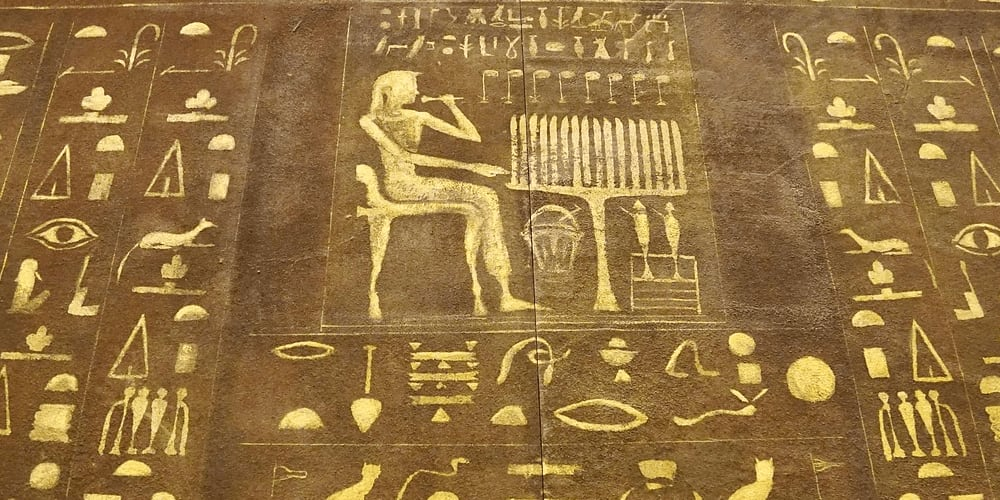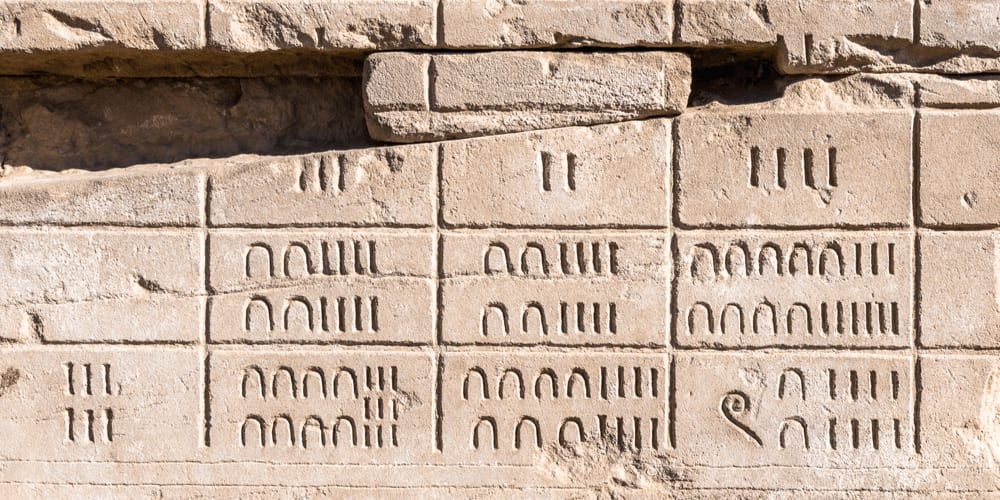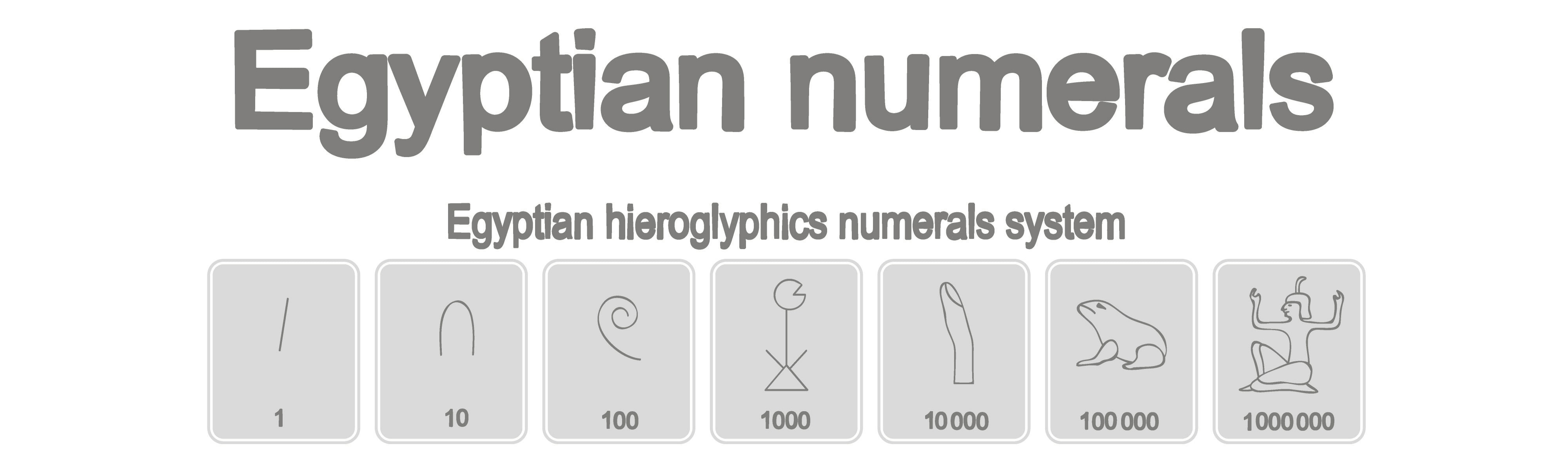# Egyptian Mathematics

The ancient Egyptians contributed a lot to the development of modern mathematical practices – learn about the creation of the decimal numeric system while reading our article on Egyptian mathematics!The earliest Egyptian settlements that were located along the Nile date back to 6000 BCE. The use of mathematical practices in Ancient Egypt was tightly connected with religion and agriculture. The Egyptians were known to record the seasons and describe the patterns of moon phases.

## System of measurement

At the dawn of their civilization, the Egyptians developed a unique system of measurement. It was only natural that the ancient units of measurement were based on body parts as the easiest way to find the size of an object is to compare it with the length of various parts of the body. Let’s explore some units of length for a better understanding of the system:• The finger, the basic unit, was about 1.875 cm
• Four fingers constituted the palm that was approximately 7.5 cm
• Seven palms constituted the cubit that was about 52.5 cm

## Numerals

According to scientific evidence, the Egyptians were the first to develop the base 10 numeration system. Again, using body parts, they created a relatively simple system that was based on ten figures. Archeologists believe that the Egyptians invented the decimal numeric system around 3000 - 2700 BCE. The Egyptians used the following hieroglyphs to represent numerals:• A stroke was used for units – one stroke meant “one,” two strokes stood for “two,” etc.
• An arch was used for tens – one arch was used for “ten,” two arches represented “twenty,” etc.
• A coiled rope was used for hundreds – one coiled rope meant “100,” while three coiled ropes were used to represent “three hundred,” etc.
• A lotus plant was used for thousands – one flower denoted “1,000,” four flowers meant “4,000,” etc.
• The number 10,000 was depicted by a finger
• A frog was used to depict the number 100,000
• A hieroglyph depicting a kneeled god with raised arms denoted 1,000,000.

## Fractions

The development of trade led to the appearance of fractions. According to archeological evidence, the ancient Egyptians introduced fractions as early as 1000 BCE. The reciprocal of a fraction was depicted by the hieroglyph “mouth.” Besides, they had special hieroglyphs to write such fractions as 1/2, 2/3, and 3/4. The use of fractions helped them to find the approximate area of a circle – they noticed that the area of a circle that has a diameter of 9 units is equivalent to the area a square of side 8 units. Therefore, to find areas of other circles they multiplied their diameter by 8/9 and then squared it. Thus, the ancient Egyptians obtained the approximate value of p.
Take a quick math quiz to test your new knowledge!
Solve math problems and have fun!
Play Quiz